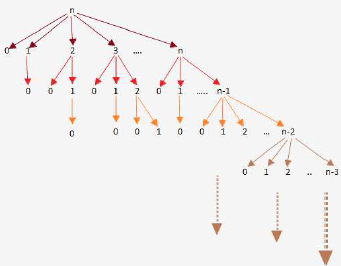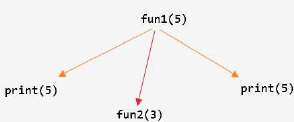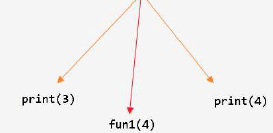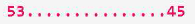Test: Recursion- 1

# Test: Recursion- 1

Test Description

## 10 Questions MCQ Test GATE Computer Science Engineering(CSE) 2023 Mock Test Series | Test: Recursion- 1

Test: Recursion- 1 for Computer Science Engineering (CSE) 2023 is part of GATE Computer Science Engineering(CSE) 2023 Mock Test Series preparation. The Test: Recursion- 1 questions and answers have been prepared according to the Computer Science Engineering (CSE) exam syllabus.The Test: Recursion- 1 MCQs are made for Computer Science Engineering (CSE) 2023 Exam. Find important definitions, questions, notes, meanings, examples, exercises, MCQs and online tests for Test: Recursion- 1 below.
Solutions of Test: Recursion- 1 questions in English are available as part of our GATE Computer Science Engineering(CSE) 2023 Mock Test Series for Computer Science Engineering (CSE) & Test: Recursion- 1 solutions in Hindi for GATE Computer Science Engineering(CSE) 2023 Mock Test Series course. Download more important topics, notes, lectures and mock test series for Computer Science Engineering (CSE) Exam by signing up for free. Attempt Test: Recursion- 1 | 10 questions in 30 minutes | Mock test for Computer Science Engineering (CSE) preparation | Free important questions MCQ to study GATE Computer Science Engineering(CSE) 2023 Mock Test Series for Computer Science Engineering (CSE) Exam | Download free PDF with solutions
 1 Crore+ students have signed up on EduRev. Have you?
Test: Recursion- 1 - Question 1

### What will be the output of the following C program? void count (int n) { static int d=1; printf ("%d",n); printf ("%d",d);  d++; if (n>1) count (n-1);  printf ("%d",d); } void main(){  count (3); }

Detailed Solution for Test: Recursion- 1 - Question 1

Here d is Static, so the value of d will persists between the function calls.

1. count(3) will print the value of n and d and increments d and call count(2) = prints 3 1.
2. count(2) will print the value of n and d and increments d and call count(1) = prints 2 2.
3. count(1) will print the value of n and d and increments d => prints 1 3.

Now it will return and prints the final incremented value of d which is 4, 3 times.
So, option A is correct = 3 1 2 2 1 3 4 4 4

Test: Recursion- 1 - Question 2

### A language with string manipulation facilities uses the following operations head(s): first character of a string tail(s): all but exclude the first character of a string concat(s1, s2): s1s2 For the string "acbc" what will be the output of concat(head(s), head(tail(tail(s))))

Detailed Solution for Test: Recursion- 1 - Question 2

concat(a,b)
ab.

Test: Recursion- 1 - Question 3

### Consider the following C function: int f(int n) { static int i = 1; if(n >= 5) return n; n = n+i;     i++; return f(n); } The value returned by f(1) is

Detailed Solution for Test: Recursion- 1 - Question 3

f(1):n=2,i=2
f(2):n=4,i=3
f(4):n=7,i=4
f(7):print(n)===>>> 7<ans>

Test: Recursion- 1 - Question 4

double foo(int n)

{

int i;

double sum;

if(n == 0)

{

return 1.0;
}

else

{

sum = 0.0;

for(i = 0; i < n; i++)

{

sum += foo(i);

}
return sum;

}
}

The space complexity of the above code is?

Detailed Solution for Test: Recursion- 1 - Question 4A. The code here is storing only local variables. So, the space complexity will be the recursion depth- maximum happening for the last iteration of the for loop- foo(n-1) - foo(n-2) - .... - foo(0) all live giving space complexity O(n).

B. To store the n values we need space complexity O(n). So, the space complexity won't change and will be O(n).

Test: Recursion- 1 - Question 5

double foo(int n)

{

int i;

double sum;

if(n == 0)

{return 1.0;

}

else

{

sum = 0.0;

for(i = 0; i < n; i++)

{

sum += foo(i);

}

return sum;

}
}

Suppose we modify the above function foo() and stores the value of foo(i) 0 <= i < n, as and when they are computed. With this modification the time complexity for function foo() is significantly reduced. The space complexity of the modified function would be:

Detailed Solution for Test: Recursion- 1 - Question 5

The modification being proposed is tabulation method of Dynamic Programming.
So let we have an auxillary array which stores the value of foo(i) once it is computed and can be used at later stage .So foo(i) is not called again , instead lookup from the table takes place. It would be something like :

#include <stdio.h>
#define max 1000
int foo[max];

foo(int n) {
if(foo[n] == -1) //compute it and store at foo[n] and return
else // return foo[n] directly
}

int main(void) {
//initialize all elements of foo[max] to -1
return 0;
}Using this approach , we need an auxillary array of size n to store foo(i) where i ranges from 0 to n-1.

So space complexity of this method = O(n) And function foo(n) computes 2n - 1

Test: Recursion- 1 - Question 6

int f(int n)

static int r = 0;

if (n <= 0) return1;

if (n > 3)

{   r = n;

return f(n-2) + 2;

}
return f(n-1) + r;

}

What is the value of f(5)?

Detailed Solution for Test: Recursion- 1 - Question 6

f(5) = 18.
f(3) + 2 = 16 + 2 = 18
f(2) + 5 = 11 + 5 = 16

f(1) + 5 = 6 + 5 = 11
f(0) + 5 = 1+5 = 6
Consider from last to first. Since it is recursive function.

Test: Recursion- 1 - Question 7

The function f is defined as follows:

int f (int n) {

if (n <= 1) return 1;

else if (n % 2 == 0) return f(n/2);

else return f(3n - 1);
}

Assuming that arbitrarily large integers can be passed as a parameter to the function, consider the following statements.

i. The function f terminates for finitely many different values of n ≥ 1.

ii. The function f terminates for infinitely many different values of n ≥ 1.

iii. The function f does not terminate for finitely many different values of n ≥ 1.

iv. The function f does not terminate for infinitely many different values of n ≥ 1.

Which one of the following options is true of the above?

A. i and iii

B. i and iv

C. ii and iii

D. ii and iv

Detailed Solution for Test: Recursion- 1 - Question 7

The function terminates for all powers of 2 (which is infinite), hence (i) is false and (ii) is TRUE.
Let n = 5.
Now, recursive calls will go like 5 - 14 - 7 - 20 - 10 - 5 -
And this goes into infinite recursion. And if we multiply 5 with any power of 2, also result will be infinite recursion. Since, there are infinite powers of 2 possible, there are infinite recursions possible (even considering this case only). So, (iv) is TRUE and (iii) is false.

Test: Recursion- 1 - Question 8

Consider the following two functions.

void fun1(int n) {

if(n == 0) return;

printf("%d", n);

fun2(n - 2);

printf("%d", n);

}

void fun2(int n) {

if(n == 0) return;

printf("%d", n);

fun1(++n);

printf("%d", n);

}

The output printed when fun1 (5) is called is

Detailed Solution for Test: Recursion- 1 - Question 8• Unroll recursion up to a point where we can distinguish the given options and choose the correct one!
• Options B and D are eliminated.
Test: Recursion- 1 - Question 9

Consider the following computation rules. Parallel-outermost rule: Replace all the outermost occurrences of F (i.e., all occurrences of F which do not occur as arguments of other F's) simultaneously. Parallel - innermost rule: Replace all the innermost occurrences of F (i.e.,all occurrences of F with all arguments free of F's) simultaneously. Now consider the evaluations of the recursive program over the integers.

F(x, y) <== if x = 0 then 0 else

[ F(x + 1, F(x, y)) * F(x - 1, F(x, y))]

where the multiplication functions * is extended as follows:

0 * w & w * 0 are 0

a * w & w * a are w (for any non-zero integer a)

w * w is w

We say that F(x, y) = w when the evaluation of F(x, y) does not terminate. Computing F(1, 0) using the parallel - innermost and parallel - outermost rule yields

Detailed Solution for Test: Recursion- 1 - Question 9

Answer is A) w and 0

If we solve using parallel innermost rule
F(1,0) = F(2,F(1,0)) * F(0,F(1,0))

=F(2, F(2,F(1,0)) * F(0,F(1,0)) ) * F(0, F(2,F(1,0)) * F(0,F(1,0)) )

Since computation of F(1,0) goes on
we assign F(1,0) to w
So F(1,0)= w

Using parallel outermost rule
F(1,0)= F(2,F(1,0)) * F(0,F(1,0))
= F(2,F(1,0)) * 0
= 0

Test: Recursion- 1 - Question 10

Consider the class of recursive and iterative programs. Which of the following is false?

Detailed Solution for Test: Recursion- 1 - Question 10

Computable function: those which can be incorporated in a program using for/while loops.

Total Function: Defined for all possible inputs
Well Defined: if its definition assigns it a unique value.

It was a belief in early 1900s that every Computable function was also Primitively Recursive. But the discovery of Ackermann function provided a counter to it.
The class of primitive recursive functions is a small subclass of the class of recursive functions. This means that there are some functions which are Well-Defined Total Functions and are Computable BUT Not primitively recursive; eg. Ackermann function.

This makes all options from option A to option D as True.
But option E as
. As iterative programs are equivalent to only Primitively Recursive class.

## GATE Computer Science Engineering(CSE) 2023 Mock Test Series

150 docs|215 tests
 Use Code STAYHOME200 and get INR 200 additional OFF Use Coupon Code
Information about Test: Recursion- 1 Page
In this test you can find the Exam questions for Test: Recursion- 1 solved & explained in the simplest way possible. Besides giving Questions and answers for Test: Recursion- 1, EduRev gives you an ample number of Online tests for practice

## GATE Computer Science Engineering(CSE) 2023 Mock Test Series

150 docs|215 tests